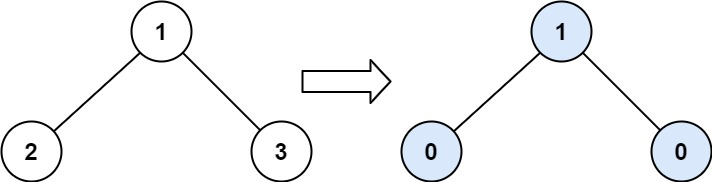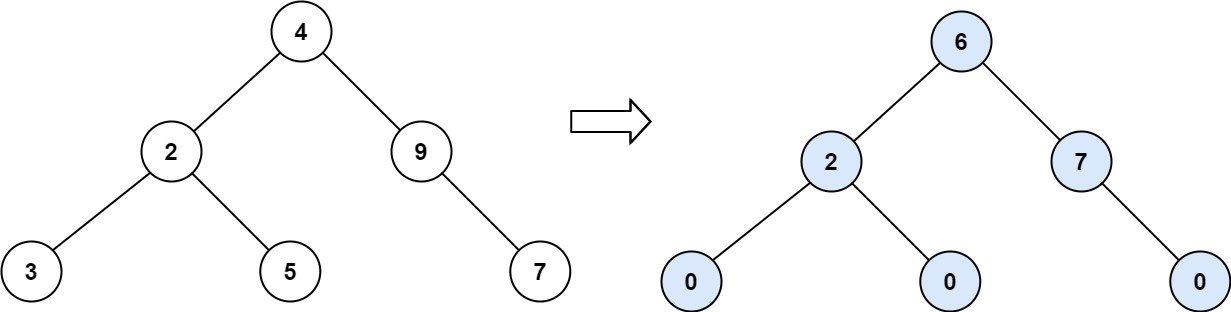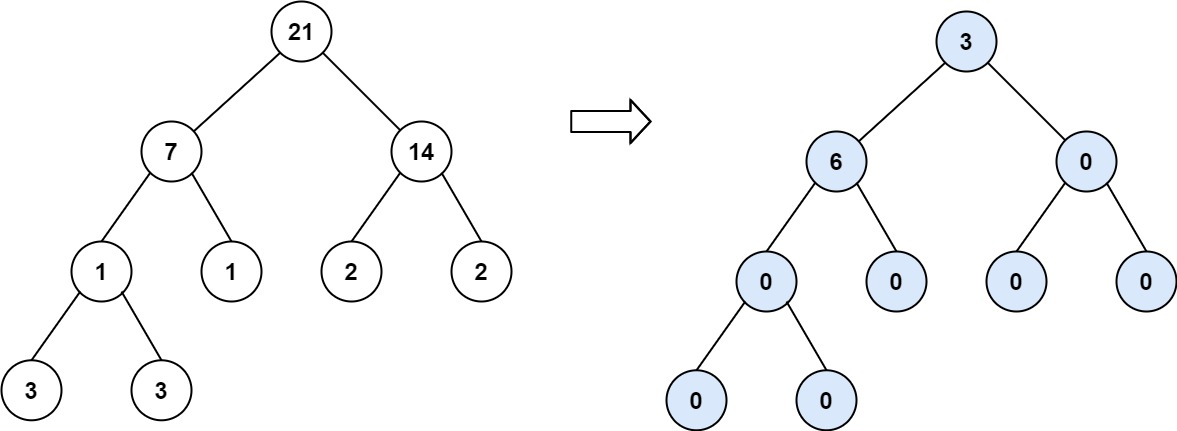Formatted question description: https://leetcode.ca/all/563.html

# 563. Binary Tree Tilt (Easy)

Given the root of a binary tree, return the sum of every tree node's tilt.

The tilt of a tree node is the absolute difference between the sum of all left subtree node values and all right subtree node values. If a node does not have a left child, then the sum of the left subtree node values is treated as 0. The rule is similar if there the node does not have a right child.

Example 1:Input: root = [1,2,3]
Output: 1
Explanation:
Tilt of node 2 : |0-0| = 0 (no children)
Tilt of node 3 : |0-0| = 0 (no children)
Tile of node 1 : |2-3| = 1 (left subtree is just left child, so sum is 2; right subtree is just right child, so sum is 3)
Sum of every tilt : 0 + 0 + 1 = 1


Example 2:Input: root = [4,2,9,3,5,null,7]
Output: 15
Explanation:
Tilt of node 3 : |0-0| = 0 (no children)
Tilt of node 5 : |0-0| = 0 (no children)
Tilt of node 7 : |0-0| = 0 (no children)
Tilt of node 2 : |3-5| = 2 (left subtree is just left child, so sum is 3; right subtree is just right child, so sum is 5)
Tilt of node 9 : |0-7| = 7 (no left child, so sum is 0; right subtree is just right child, so sum is 7)
Tilt of node 4 : |(3+5+2)-(9+7)| = |10-16| = 6 (left subtree values are 3, 5, and 2, which sums to 10; right subtree values are 9 and 7, which sums to 16)
Sum of every tilt : 0 + 0 + 0 + 2 + 7 + 6 = 15


Example 3:Input: root = [21,7,14,1,1,2,2,3,3]
Output: 9


Constraints:

• The number of nodes in the tree is in the range [0, 104].
• -1000 <= Node.val <= 1000

Related Topics:
Tree, Depth-first Search, Recursion

Similar Questions:

## Solution 1.

// OJ: https://leetcode.com/problems/binary-tree-tilt/
// Time: O(N)
// Space: O(H)
class Solution {
int ans = 0;
int dfs(TreeNode *root) {
if (!root) return 0;
int L = dfs(root->left), R = dfs(root->right);
ans += abs(L - R);
return L + R + root->val;
}
public:
int findTilt(TreeNode* root) {
dfs(root);
return ans;
}
};


Java

• /**
* Definition for a binary tree node.
* public class TreeNode {
*     int val;
*     TreeNode left;
*     TreeNode right;
*     TreeNode(int x) { val = x; }
* }
*/
class Solution {
public int findTilt(TreeNode root) {
if (root == null)
return 0;
int leftTilt = findTilt(root.left), rightTilt = findTilt(root.right);
int leftSum = nodeValuesSum(root.left), rightSum = nodeValuesSum(root.right);
int rootTilt = Math.abs(leftSum - rightSum);
return leftTilt + rightTilt + rootTilt;
}

public int nodeValuesSum(TreeNode root) {
if (root == null)
return 0;
else
return root.val + nodeValuesSum(root.left) + nodeValuesSum(root.right);
}
}

• // OJ: https://leetcode.com/problems/binary-tree-tilt/
// Time: O(N)
// Space: O(H)
class Solution {
int ans = 0;
int dfs(TreeNode *root) {
if (!root) return 0;
int L = dfs(root->left), R = dfs(root->right);
ans += abs(L - R);
return L + R + root->val;
}
public:
int findTilt(TreeNode* root) {
dfs(root);
return ans;
}
};

• # Definition for a binary tree node.
# class TreeNode(object):
#     def __init__(self, x):
#         self.val = x
#         self.left = None
#         self.right = None

class Solution(object):
def findTilt(self, root):
"""
:type root: TreeNode
:rtype: int
"""

def dfs(root):
if not root:
return 0, 0  # sum, tilt sum

leftSum, leftTilt = dfs(root.left)
rightSum, rightTilt = dfs(root.right)

return leftSum + root.val + rightSum, abs(leftSum - rightSum) + leftTilt + rightTilt

return dfs(root)